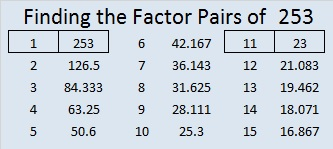# 253 and Level 1

• 253 is a composite number.
• Prime factorization: 253 = 11 x 23
• The exponents in the prime factorization are 1 and 1. Adding one to each and multiplying we get (1 + 1)(1 + 1) = 2 x 2 = 4. Therefore 253 has 4 factors.
• Factors of 253: 1, 11, 23, 253
• Factor pairs: 253 = 1 x 253 or 11 x 23
• 253 has no square factors that allow its square root to be simplified. √253 ≈ 15.906Print the puzzles or type the factors on this excel file: 12 Factors 2014-10-06